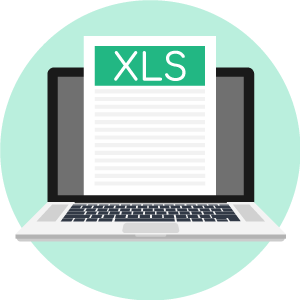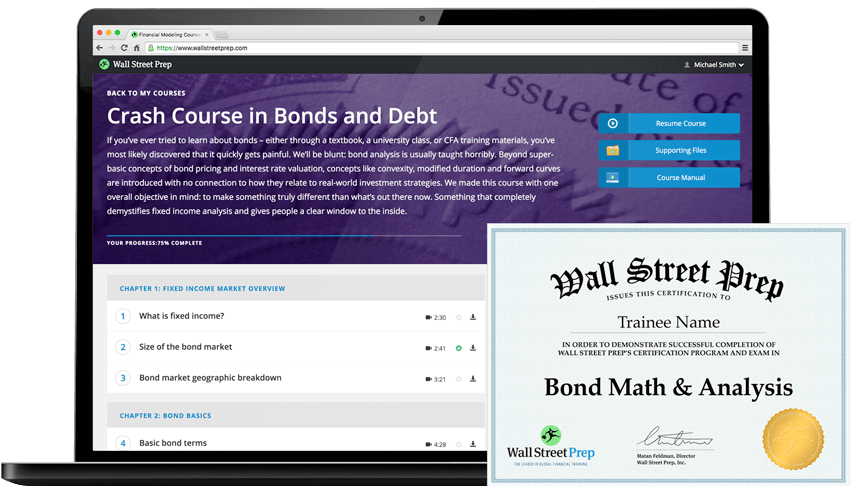# Yield to Maturity (YTM)

Guide to Understanding the Concept of Yield to Maturity (YTM)• What are the steps to calculating the yield to maturity (YTM) in Excel?
• Which factors determine the yield to maturity (YTM) on a bond?
• What is the relationship between a bond’s price and its yield to maturity (YTM)?
• How does the YTM differ from the current yield?

## Yield to Maturity (YTM) Definition

One of the most frequently used metrics for evaluating potential investments by bond and fixed income investors is the yield to maturity (YTM).

The YTM is the estimated annual rate of return that a bond is expected to earn until reaching maturity, with three notable assumptions:

1. The return assumes the bond investor held onto the debt instrument until the maturity date.
2. All the required interest payments and principal repayment were made on schedule.
3. The coupon payments were reinvested at the same rate as the yield-to-maturity (YTM).

Said differently, the yield to maturity (YTM) on a bond is its internal rate of return (IRR) – i.e. the discount rate which makes the present value (PV) of all the bond’s future cash flows equal to its current market price.

## Yield to Maturity (YTM) FormulaThe calculations in the yield to maturity (YTM) formula consist of the following factors:

• Coupon Rate (C): Often referred to as the bond’s interest rate, the coupon rate is the periodic payments made regularly from the bond issuer to the investors – in general, the higher the coupon rate attached to the bond, the higher the yield, all else being equal.
• Face Value (FV): The face value of a bond (i.e. the par value) is the amount to be repaid to a bondholder on the date of maturity.
• Present Value (PV): The present value (PV) of the bond refers to the current market price and how much investors are willing to pay for the bond in the open market as of the present date, which may be higher (or lower) than the bond’s FV based on the market conditions and supply/demand.
• Maturity Date: The pre-specified date on which the issuer is contractually obligated to repay the principal – from this date, the number of years to maturity can be derived.
• Number of Compounding Periods (n): The number of compounding periods refers to the number of payments made in one year multiplied by the number of years to maturity (e.g. five years until maturity and semi-annual coupon payments would mean n = 10 periods).

## Importance of Yield to Maturity

The yield of maturity (YTM) metric facilitates comparisons among different bonds and their expected returns, which helps investors make more informed decisions on how to manage their bond portfolios.

Even for bonds consisting of different maturities and coupon rates, the YTM enables comparisons to be made since the YTM is expressed as an annualized rate regardless of the bond’s years to maturity.

By understanding the YTM formula, investors can better predict how changing market conditions could impact their portfolio holdings based on their portfolio strategy and existing investments.

Considering yields rise when prices drop (and vice versa), investors can project the yield-to-maturity (YTM) on portfolio investments to guide better decision-making.

The YTM can also enable debt investors to assess their degree of exposure to interest rate risk, which is defined as the potential downside caused by sudden changes in interest rates.

The relationship between the current YTM and interest rate risk is inversely proportional, which means the higher the YTM, the less sensitive the bond prices are to interest rate changes.

## Yield to Maturity (YTM) vs Current Yield

The yield to maturity (YTM), as mentioned earlier, is the annualized return on a debt instrument based on the total payments received from the date of initial purchase until the maturation date.

In comparison, the current yield on a bond is the annual coupon income divided by the current price of the bond security.

An important distinction between a bond’s YTM and its coupon rate is the YTM fluctuates over time based on the prevailing interest rate environment, whereas the coupon rate is fixed.

###### Yield to Maturity (YTM) and Coupon Rate / Current Yield
• If the YTM < Coupon Rate and Current Yield → The bond is being sold at a “premium” to its par value.
• If the YTM > Coupon Rate and Current Yield → The bond is being sold at a “discount” to its par value.
• If the YTM = Coupon Rate and Current Yield → The bond is said to be “trading at par”.

## Drawbacks to Yield to Maturity (YTM)

The most noteworthy drawback to the yield to maturity (YTM) measure is that YTM does NOT account for a bond’s reinvestment risk.

Simply put, the bond’s coupon payments are assumed to be reinvested at the same rate as the YTM, which may not be an option in the future given uncertainties regarding the markets.

In effect, if coupons were to be reinvested at lower rates than the YTM, the calculated YTM is going to turn out to have been inaccurate, as the return on the bond would have been overstated.

Additionally, the standard YTM formula is meant to be an approximation as opposed to being a precise figure – for instance, the YTM is prone to error due to the potential for unexpected events such as if the bondholder decides not to reinvest all coupon payments or if the bond is called early (i.e. repaid prior to the maturity date).

However, the benefits related to comparability tend to outweigh the drawbacks, which explains the widespread usage of YTM across the debt markets and fixed-income investors.

Yet, the YTM’s assumptions that all coupon payments are made as scheduled and that interest is reinvested at the same rate are nonetheless risky, simplified assumptions.

## Yield to Maturity (YTM) Excel Template

Now that we’ve explained the concept behind yield-to-maturity (YTM), we’re ready to practice calculating this metric in Excel.Submitting ...

## Yield to Maturity (YTM) Model Assumptions

In our hypothetical scenario, the following assumptions regarding the bond will be used to calculate the yield-to-maturity (YTM).

###### Model Inputs
• Face Value of Bond (FV): \$1,000
• Annual Coupon Rate (%): 6.0%
• Number of Years to Maturity: 10 Years
• Price of Bond (PV): \$1,050

We’ll also assume that the bond issues semi-annual coupon payments.

Given those inputs, the next step is to calculate the semi-annual coupon rate, which we can calculate by dividing the annual coupon rate by two.

• Semi-Annual Coupon Rate (%) = 6.0% ÷ 2 = 3.0%

Then, we must calculate the number of compounding periods by multiplying the number of years to maturity by the number of payments made per year.

• Number of Compounding Periods (n) = 10 × 2 = 20

As for our last input, we multiply the semi-annual coupon rate by the face value of the bond (FV) to arrive at the annual coupon of the bond.

• Annual Coupon (C) = 3.0% × \$1,000 = \$30## Yield to Maturity (YTM) Example Calculation

With all required inputs complete, we can calculate the semi-annual yield to maturity (YTM).

• Semi-Annual Yield-to-Maturity (YTM) = [\$30 + (\$1,000 – \$1,050) / 20] / [(\$1,000 + \$1,050) / 2]
• Semi-Annual YTM = 2.7%

Now, for the final step, we must convert our semi-annual YTM to an annual percentage rate – i.e. the annualized yield to maturity (YTM).

• Annual Yield to Maturity (YTM) = 2.7% × 2 = 5.4%#### Crash Course in Bonds and Debt: 8+ Hours of Step-By-Step Video

A step-by-step course designed for those pursuing a career in fixed income research, investments, sales and trading or investment banking (debt capital markets).Inline FeedbacksLearn Online: Crash Course in Bonds

For those pursuing fixed income research, investments, sales and trading or investment banking.

X

The Wall Street Prep Quicklesson Series

7 Free Financial Modeling Lessons

Get instant access to video lessons taught by experienced investment bankers. Learn financial statement modeling, DCF, M&A, LBO, Comps and Excel shortcuts.